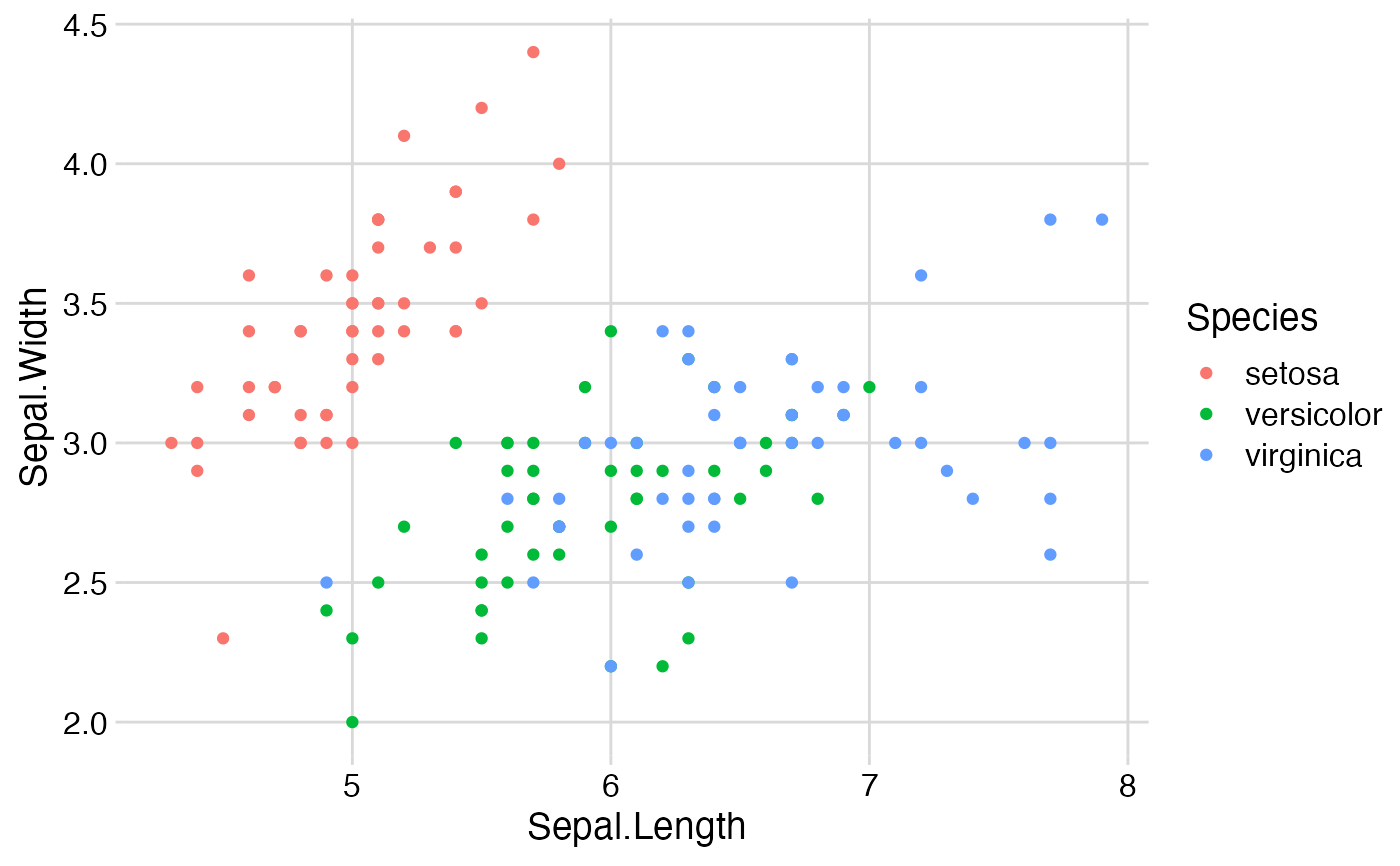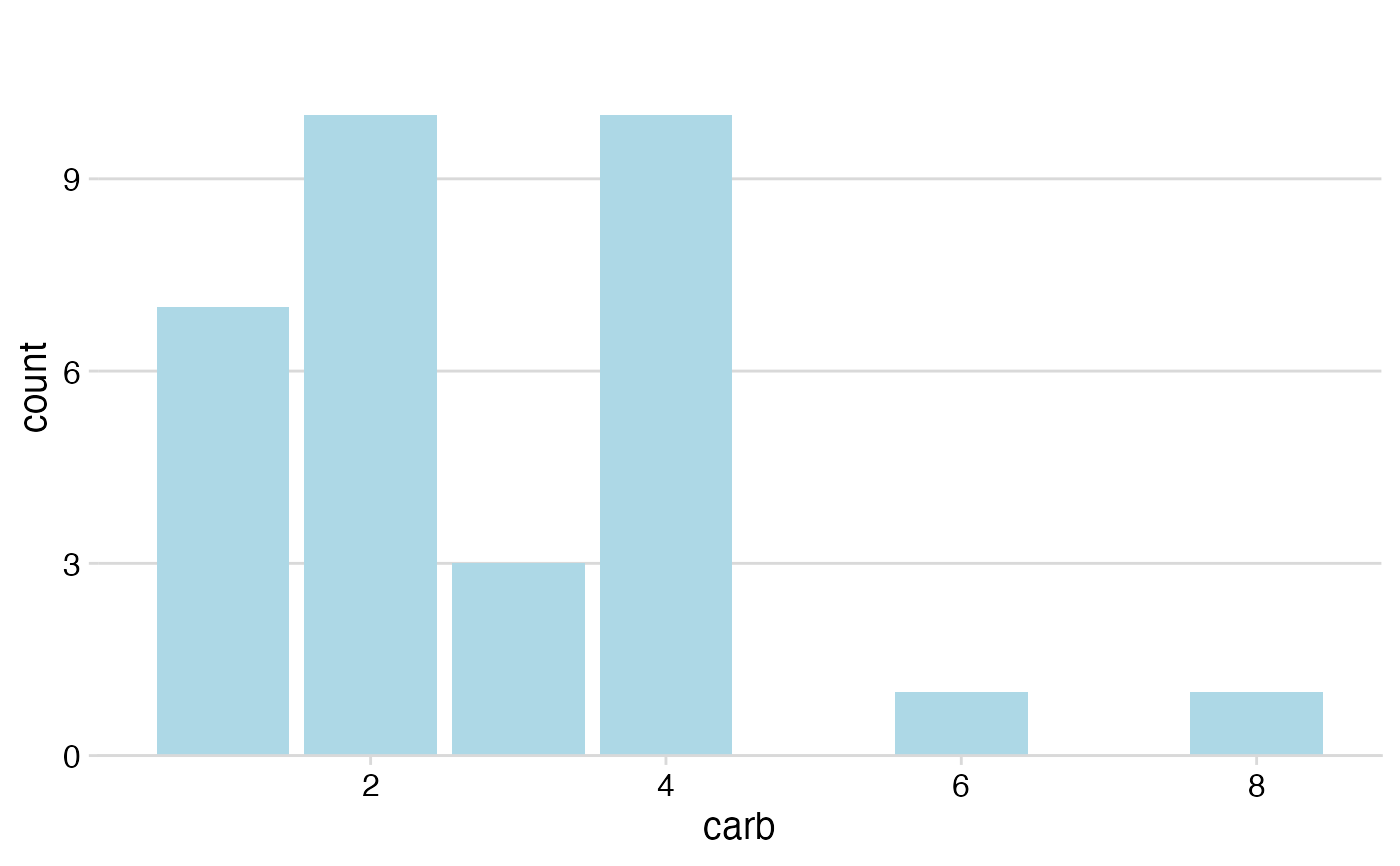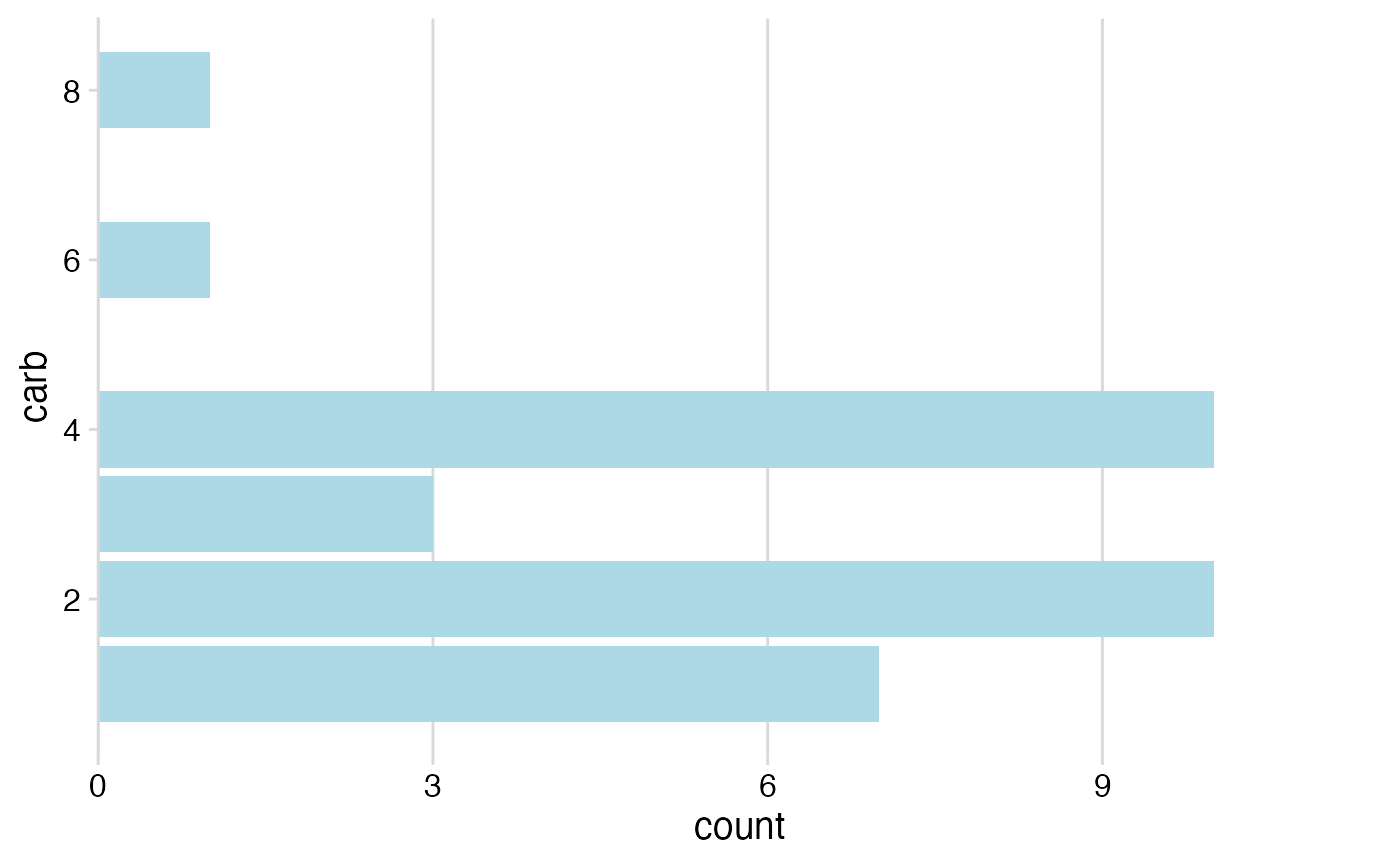Three minimalistic themes that provide either a full grid, a horizontal grid, or a vertical grid. Similar to theme_minimal(), but with some stylistic differences. Most importantly, these themes do not draw minor grid lines. Also, font sizes are coordinated with theme_half_open() and with the defaults in the save_plot() function.

theme_minimal_grid(
font_size = 14,
font_family = "",
line_size = 0.5,
rel_small = 12/14,
rel_tiny = 11/14,
rel_large = 16/14,
color = "grey85",
colour
)

theme_minimal_vgrid(
font_size = 14,
font_family = "",
line_size = 0.5,
rel_small = 12/14,
rel_tiny = 11/14,
rel_large = 16/14,
color = "grey85",
colour
)

theme_minimal_hgrid(
font_size = 14,
font_family = "",
line_size = 0.5,
rel_small = 12/14,
rel_tiny = 11/14,
rel_large = 16/14,
color = "grey85",
colour
)

## Arguments

font_size Overall font size. Font family for plot title, axis titles and labels, legend texts, etc. Line size for grid lines. Relative size of small text (e.g., axis tick labels) Relative size of tiny text (e.g., caption) Relative size of large text (e.g., title) Color of grid lines.

## Details

theme_minimal_grid() provides a minimal grid theme. theme_minimal_hgrid() strips down this theme even further and draws only horizontal lines, and theme_minimal_vgrid() does the same for vertical lines.

## Examples

library(ggplot2)

# theme_minimal_grid()
ggplot(iris, aes(x = Sepal.Length, y = Sepal.Width, color = Species)) +
geom_point() + theme_minimal_grid()# theme_minimal_hgrid()
ggplot(mtcars, aes(x = carb)) +
geom_bar(fill = "lightblue") +
scale_y_continuous(limits = c(0, 11.5), expand = c(0, 0)) +
theme_minimal_hgrid()# theme_minimal_vgrid()
ggplot(mtcars, aes(x = carb)) +
geom_bar(fill = "lightblue") +
scale_y_continuous(limits = c(0, 11.5), expand = c(0, 0)) +
coord_flip() +
theme_minimal_vgrid()